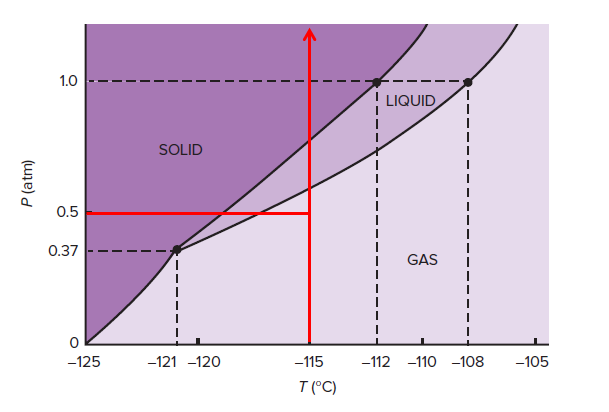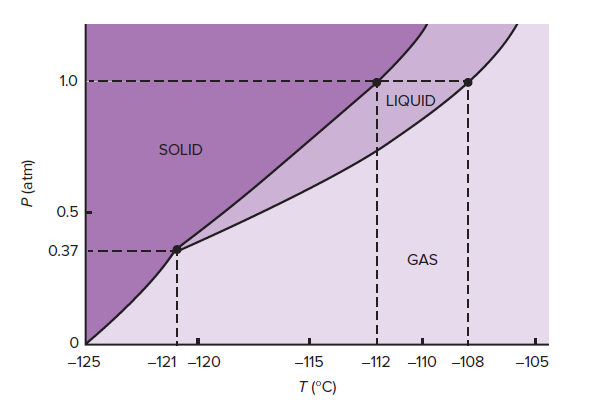# Problem: The phase diagram for xenon is shown below.(b) Describe the phase changes that a sample of xenon undergoes at −115°C as it is compressed from 0.5 atm to 25 atm. (The critical pressure of xenon is 58 atm.)

###### FREE Expert Solution83% (187 ratings)###### Problem Details

The phase diagram for xenon is shown below.

(b) Describe the phase changes that a sample of xenon undergoes at −115°C as it is compressed from 0.5 atm to 25 atm. (The critical pressure of xenon is 58 atm.)